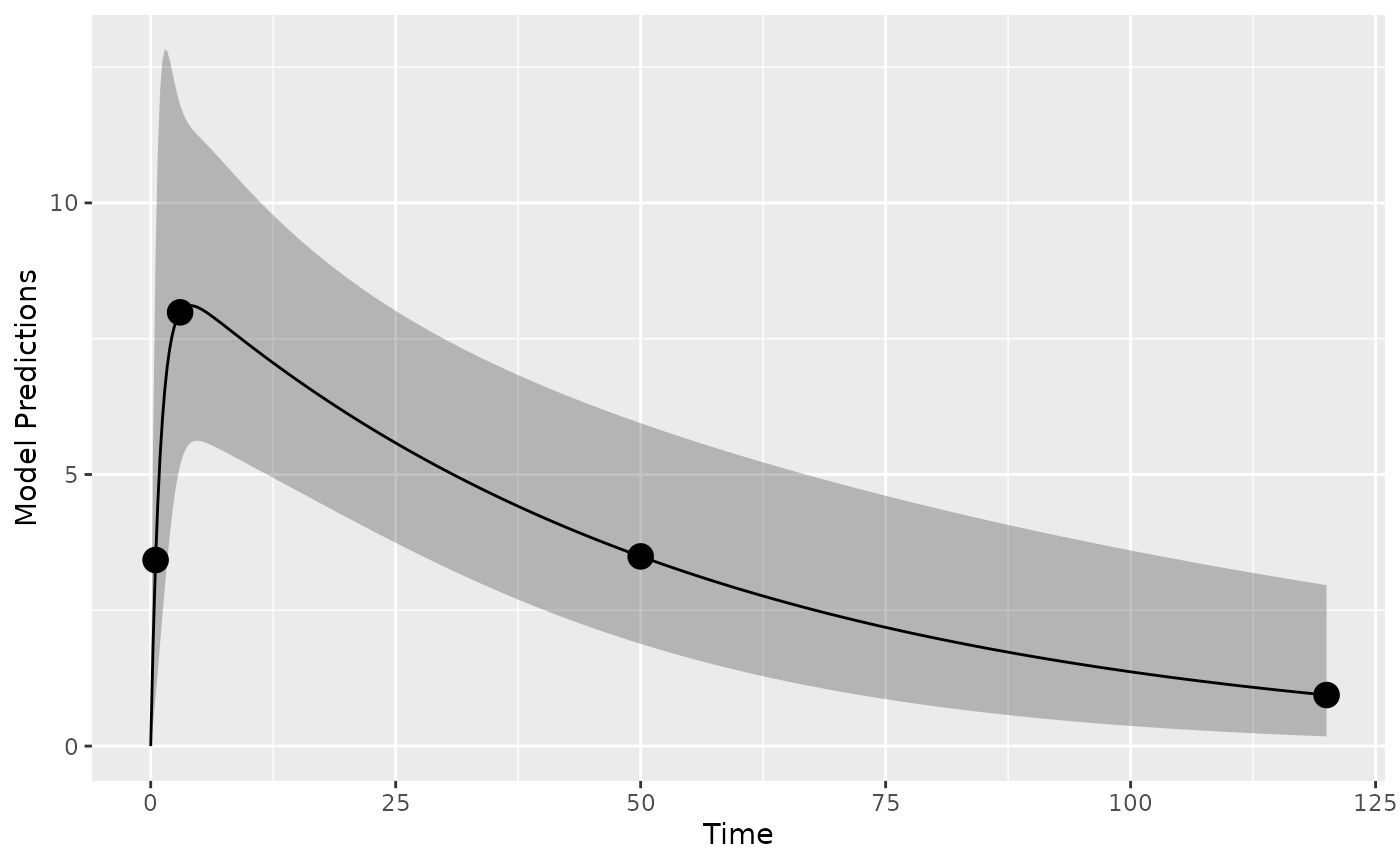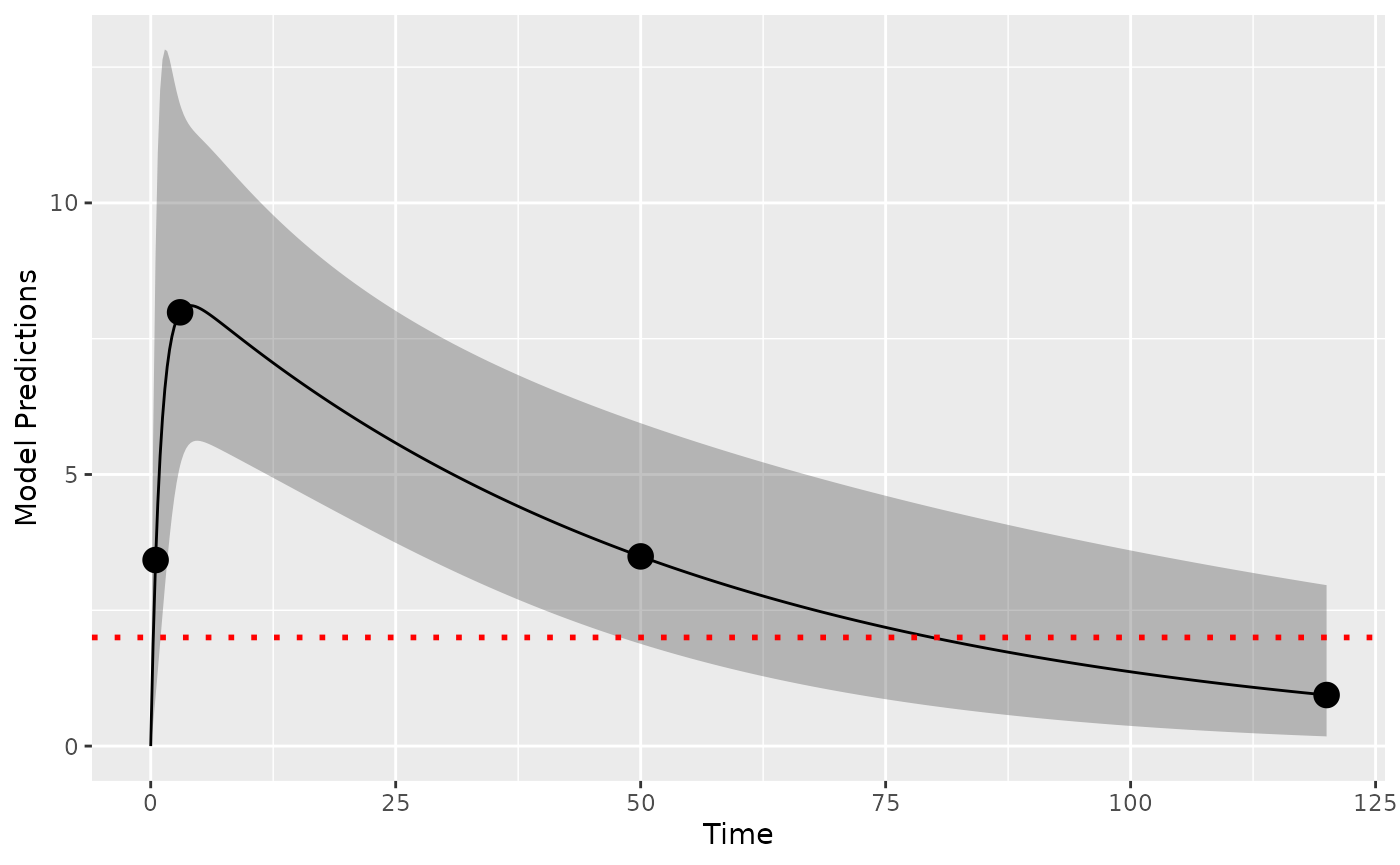library(PopED)
packageVersion("PopED")
#>  '0.6.0'

# Define a model

Here we define, as an example, a one-compartment pharmacokinetic model with linear absorption (analytic solution) in PopED .

ff <- function(model_switch,xt,parameters,poped.db){
with(as.list(parameters),{
y=xt
y=(DOSE*KA/(V*(KA-CL/V)))*(exp(-CL/V*xt)-exp(-KA*xt))
return(list(y=y,poped.db=poped.db))
})}

Next we define the parameters of this function. DOSEis defined as a covariate (in vector a) so that we can optimize the value later.

sfg <- function(x,a,bpop,b,bocc){
parameters=c( CL=bpop*exp(b),
V=bpop*exp(b),
KA=bpop*exp(b),
DOSE=a)
}

We will use an additive and proportional residual unexplained variability (RUV) model, predefined in PopED as the function feps.add.prop.

# Define an initial design and design space

Now we define the model parameter values, the initial design and design space for optimization.

We define model parameters similar to the Warfarin example from the software comparison in and an arbitrary design of two groups of 20 individuals.

poped_db <-
create.poped.database(
ff_fun=ff,
fg_fun=sfg,
bpop=c(CL=0.15, V=8, KA=1.0),
d=c(CL=0.07, V=0.02, KA=0.6),
groupsize=32,
xt=c( 0.5,3,50,120),
discrete_xt = list(c(0.5,1:120)),
minxt=0,
maxxt=120,
a=70,
mina=0,
maxa=100)


# Simulation

First it may make sense to check your model and design to make sure you get what you expect when simulating data. Here we plot the model typical values for the two groups:

plot_model_prediction(poped_db, model_num_points = 500,facet_scales = "free",PI=T)# Design evaluation

Next, we evaluate the initial design. We see that the relative standard error of the parameters (in percent) are relatively well estimated with this design except for the proportional RUV.

eval_full <- evaluate_design(poped_db)
Expected parameter RSE (in %) for the initial design.
RSE
CL 5
V 4
KA 15
d_CL 34
d_V 70
d_KA 28
sig_prop 89

# LOQ handling

We assume that the LOQ level is at 2 concentration units.

library(ggplot2)
plot_model_prediction(poped_db, model_num_points = 500,facet_scales = "free",PI=T) +
geom_hline(yintercept = 2,color="red",linetype="dotted")We use optimization criteria based on the D6 (loq_method=1 which is the default) and D2 (loq_method=2) methods from . For the D6 method, which has been shown to be a better method in comparison to SSE studies, we have updated the method to only investigate points where the 95% PI overlaps LOQ, otherwise we set the design point to either no information or full information. Further we filter out situations with very low probabilities (loq_prob_limit = 0.001). Both the CI% and the low probability limit can be specified in the calculation (default values are loq_PI_conf_level = 0.95 and loq_prob_limit = 0.001). In this way we can get the D6 method to compute in reasonable time-frames even for larger number of design samples.

Here we can evaluate the design with both methods and test the speed of computation. We see that D6 is significantly slower than D2 (but D6 should be a more accurate representation of the RSE expected using M3 estimation methods).

set.seed(1234)
e_time_D6 <- system.time(
eval_D6 <- evaluate_design(poped_db,loq=2)
)

e_time_D2 <- system.time(
eval_D2 <- evaluate_design(poped_db,loq=2, loq_method=2)
)

cat("D6 evaluation time: ",e_time_D6,"\n" )
cat("D2 evaluation time: ",e_time_D2,"\n" )
#> D6 evaluation time:  0.367
#> D2 evaluation time:  0.135

The D2 nmethod is the same as removing the last data point

poped_db_2 <- create.poped.database(
ff_fun=ff,
fg_fun=sfg,
bpop=c(CL=0.15, V=8, KA=1.0),
d=c(CL=0.07, V=0.02, KA=0.6),
groupsize=32,
xt=c( 0.5,3,50),
discrete_xt = list(c(0.5,1:120)),
minxt=0,
maxxt=120,
a=70,
mina=0,
maxa=100)
eval_red <- evaluate_design(poped_db_2)
testthat::expect_equal(eval_red$ofv,eval_D2$ofv)
testthat::expect_equal(eval_red$rse,eval_D2$rse)

Predicted parameter uncertainty for the three methods is shown below. We see that the uncertainty is generally higher with the LOQ evaluations (as expected). We also see that because the D2 method ignores data that is below LOQ (the last observation in the design), then the predictions of uncertainty are significantly larger.

RSE (in %) for the initial design using different methods of handling LOQ.
Parameter No LOQ D6 D2
CL 5 6 6
V 4 4 4
KA 15 17 15
d_CL 34 50 498
d_V 70 109 428
d_KA 28 33 113
sig_prop 89 161 1444

## ULOQ handling

If needed we can also handle upper limits of quantification. Lets assume we have an ULOQ at 7 units in addition to the LLOQ of 2 units:

library(ggplot2)
plot_model_prediction(poped_db, model_num_points = 500,facet_scales = "free",
PI=T, PI_alpha = 0.1) +
geom_hline(yintercept = 2,color="red",linetype="dotted") +
geom_hline(yintercept = 7,color="blue",linetype="dotted")eval_ul_D6 <-evaluate_design(poped_db,
loq=2,
uloq=7)

eval_ul_D2 <- evaluate_design(poped_db,
loq=2,
loq_method=2,
uloq=7,
uloq_method=2)
#> Problems inverting the matrix. Results could be misleading.
RSE (in %) for the initial design using different methods of handling LOQ and ULOQ.
Parameter No LOQ D6 (only LLOQ) D2 (only LLOQ) D6 (ULOQ and LLOQ) D2 (ULOQ and LLOQ)
CL 5 6 6 6 6
V 4 4 4 8 0
KA 15 17 15 21 14
d_CL 34 50 498 59 276
d_V 70 109 428 203 1743
d_KA 28 33 113 35 55
sig_prop 89 161 1444 297 1645
sig_add 36 118 2127 122 6

# Design optimization

We can then optimize the design using the different methods of computing the FIM. Here we optimize only using lower LOQ.

optim_D6 <- poped_optim(poped_db, opt_xt = TRUE,
parallel=T,
loq=2)

optim_D2 <- poped_optim(poped_db, opt_xt = TRUE,
parallel=T,
loq=2,
loq_method=2)

optim_full <- poped_optim(poped_db, opt_xt = TRUE,
parallel=T)
All designs together in one plot show how the different handling of BLQ data results in different optimal designs.Design points for the apraoch ignoring LOQ, using the D2 method, and using the D6 method

Predictions using the D6 method from each of the optimizations shows the expected %RSE of the parameters if each design is used and the LOQ is at 2 concentration units. We see that D2 may be a reasonable strategy to optimize designs that are “good enough” if the D6 method is too slow for optimization.

optim_full_D6<- with(optim_full,
evaluate_design(poped.db,
loq=2))

optim_D2_D6<- with(optim_D2,
evaluate_design(poped.db,
loq=2))

optim_D6_D6<- with(optim_D6,
evaluate_design(poped.db,
loq=2))
RSE (in %) for the optimized designs evaluated using the D6 method.
Parameter No LOQ D6 D2
CL 7 5 6
V 4 3 3
KA 16 17 16
d_CL 67 34 43
d_V 58 54 52
d_KA 30 39 30
sig_prop 114 96 93

# Version information

sessionInfo()
#> R version 4.1.0 (2021-05-18)
#> Platform: x86_64-apple-darwin17.0 (64-bit)
#> Running under: macOS Big Sur 10.16
#>
#> Matrix products: default
#> BLAS:   /Library/Frameworks/R.framework/Versions/4.1/Resources/lib/libRblas.dylib
#> LAPACK: /Library/Frameworks/R.framework/Versions/4.1/Resources/lib/libRlapack.dylib
#>
#> locale:
#>  en_US.UTF-8/en_US.UTF-8/en_US.UTF-8/C/en_US.UTF-8/en_US.UTF-8
#>
#> attached base packages:
#>  stats     graphics  grDevices utils     datasets  methods   base
#>
#> other attached packages:
#>  kableExtra_1.3.4 knitr_1.33       ggplot2_3.3.3    PopED_0.6.0
#>  testthat_3.0.2
#>
#> loaded via a namespace (and not attached):
#>   svglite_2.0.0     mvtnorm_1.1-1     prettyunits_1.1.1 ps_1.6.0
#>   gtools_3.8.2      assertthat_0.2.1  rprojroot_2.0.2   digest_0.6.27
#>   utf8_1.2.1        R6_2.5.0          evaluate_0.14     highr_0.9
#>  httr_1.4.2        pillar_1.6.1      rlang_0.4.11      rstudioapi_0.13
#>  callr_3.7.0       jquerylib_0.1.4   rmarkdown_2.8     pkgdown_1.6.1
#>  labeling_0.4.2    textshaping_0.3.4 desc_1.3.0        devtools_2.4.1
#>  webshot_0.5.2     stringr_1.4.0     munsell_0.5.0     compiler_4.1.0
#>  xfun_0.23         pkgconfig_2.0.3   systemfonts_1.0.2 pkgbuild_1.2.0
#>  htmltools_0.5.1.1 tidyselect_1.1.1  tibble_3.1.2      bookdown_0.22
#>  codetools_0.2-18  viridisLite_0.4.0 fansi_0.4.2       crayon_1.4.1
#>  dplyr_1.0.6       withr_2.4.2       MASS_7.3-54       grid_4.1.0
#>  jsonlite_1.7.2    gtable_0.3.0      lifecycle_1.0.0   DBI_1.1.1
#>  magrittr_2.0.1    scales_1.1.1      cli_2.5.0         stringi_1.6.2
#>  cachem_1.0.5      farver_2.1.0      fs_1.5.0          remotes_2.3.0
#>  xml2_1.3.2        bslib_0.2.5.1     ellipsis_0.3.2    ragg_1.1.2
#>  vctrs_0.3.8       generics_0.1.0    boot_1.3-28       tools_4.1.0
#>  glue_1.4.2        purrr_0.3.4       processx_3.5.2    pkgload_1.2.1
#>  fastmap_1.1.0     yaml_2.2.1        colorspace_2.0-1  sessioninfo_1.1.1
#>  rvest_1.0.0       memoise_2.0.0     sass_0.4.0        usethis_2.0.1